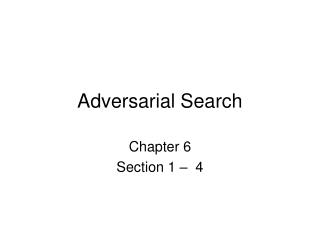# Adversarial Search - PowerPoint PPT PresentationDownload PresentationDownload Presentation- - - - - - - - - - - - - - - - - - - - - - - - - - - E N D - - - - - - - - - - - - - - - - - - - - - - - - - - -
##### Presentation Transcript

1. Adversarial Search Chapter 6 Section 1 – 4

2. Games vs. search problems • "Unpredictable" opponent  specifying a move for every possible opponent reply • Time limits  unlikely to find goal, must approximate • Solutions • Search problems: a path • Games: a strategy • But how?

3. Game tree (2-player, deterministic, turns)

4. Minimax • Perfect play for deterministic games • Idea: choose move to position with highest minimax value = best achievable payoff against best play • E.g., 2-ply game:

5. Minimax algorithm

6. Properties of minimax • Complete? Yes (if tree is finite) • Optimal? Yes (against an optimal opponent) • Time complexity? O(bm) • Space complexity? O(bm) (depth-first exploration) • For chess, b ≈ 35, m ≈100 for "reasonable" games exact solution completely infeasible

7. α-β pruning example

8. α-β pruning example

9. α-β pruning example

10. α-β pruning example

11. α-β pruning example

12. Properties of α-β • Pruning does not affect final result • Good move ordering improves effectiveness of pruning • With "perfect ordering," time complexity = O(bm/2) • A simple example of the value of reasoning about which computations are relevant (a form of meta-reasoning)

13. Resource limits Suppose we have 100 secs, explore 104 nodes/sec106nodes per move Standard approach: • cutoff test: e.g., depth limit (perhaps add quiescence search) Explore nonquiescent positions further until quiescence • evaluation function = estimated desirability of position

14. Evaluation functions • For chess, typically linear weighted sum of features Eval(s) = w1 f1(s) + w2 f2(s) + … + wn fn(s) • e.g., w1 = 9 with f1(s) = (number of white queens) – (number of black queens), etc.

15. Cutting off search MinimaxCutoff is identical to MinimaxValue except • Terminal? is replaced by Cutoff? • Utility is replaced by Eval Does it work in practice? bm = 106, b=35  m=4 4-step lookahead is a hopeless chess player! • 4-step ≈ human novice • 8-step ≈ typical PC, human master • 12-step ≈ Deep Blue, Kasparov